Categories

## So many skills involved: 0.2(d – 6) = 0.3d + 5 – 3 + 0.1d

This is a very challenging set of student work to look at as a teacher. It’s tempting to just mark this up as “confused about how to deal with complex equations,” but just look at all the moving parts! There are so many things going on here, and so many things that all of these kids know how to do.

For example, each of these kids distributes — not entirely correctly, but they do distribute.

In particular, not every kid handles the sign correctly — a bunch of kids are during 0.2(d – 6) into 0.2d + something. That’s interesting, and a discrete algebraic move that we could focus on later.

And then there is the multiplication of 0.2 and 6. Unclear whether that’s a mistake these kids would make under calmer situations.

Every kid knows they’re trying to get all the variable expressions on one side, though some kids subtract or move the 0.4d rather than subtract it from each side. (It makes me wonder if they would’ve made the same mistake if the 0.2d was on the right side, as then it would be subtracting a smaller thing from a larger thing. Kids often have a harder time seeing subtraction if it involves creating a negative quantity.)

So much complexity here! I’m sure there’s more that I haven’t noticed.

Thanks Deb!

Categories

## Solving equations, trying to distribute, lots of stuff going on!What does this student already know? In other words, what don’t you have to teach again?

What’s going on with the distribution?

You’ve got time for a 5 minute activity to help this student. What activity do you pick?

(Thanks Shauna!)

Categories

## What makes an equation tricky to solve?

Here are four equations, each using similar numbers. Which one would be the most difficult for a student to solve?Conventional wisdom is that this question is too complex to really grapple with; it depends on the kids, the class, what they’re studying, etc.

I think the conventional wisdom has a point, but I know that I’ve learned a lot about the differences between these four problems, and there ought to be a way to teach what I’ve learned to others. Of course, only other people can be the judge of whether I know something worth teaching! Here’s a rough attempt to get at some of the pedagogical structure that underlies these problems.

## Equations that can trigger arithmetic

Part of the problem with trying to make generalizations about how kids will tend to struggle or succeed in solving equations is that there are MANY ways to successfully solve an equation. I can’t say, “when kids see bla bla bla they do bla bla bla,” because just as many kids will as won’t do that, and it largely depends on the approaches to instruction that the teacher takes with the class.

So I want to play with the idea that it can be helpful to think of an equation as having triggers that can activate various strategies that a kid might potentially use for an equation.

Here’s at attempt to explain what I mean in the context of the four equations above. There are many different ways that a child might think about solving x – 12 = 9, especially after receiving instruction on how to solve these types of equations from a teacher. Here are just a few:

• An “undoing” metaphor which involves adding 12 to the right side
• A “balanced moves” metaphor which would involve adding 12 to both sides
• Just to get weird: a kid could multiply both sides by -1, add x to both sides, then perform 12 – (-9).

I’m sure there are many more potential strategies out there. What I think is special about x – 12 = 9, though, is that it is especially well-suited for one way of thinking, one strategy, in particular:

• Just treat it like an arithmetic problem — what minus 12 is 9?

The idea I’m playing with is that the equation contains a trigger for using an arithmetic strategy, i.e. a strategy that depends on no new algebraic metaphors, procedures or ideas, just whatever knowledge of the operations and arithmetic a student comes into this work with.

I want to be clear about two things. First, I’m not claiming that all students are going to approach this equation using arithmetic. Instead, I’m saying that these equations are especially well-suited for arithmetic, and a student who uses arithmetic sometimes to solve equations would very often use it in x – 12 = 9.

Second, I think that arithmetic is generally something that students have had a lot of experience in, compared to algebra. By the time an 8th Grader gets to an equation like x – 12 = 9, thinking about this equation in terms of arithmetic is likely to be productive and yield correct and helpful thoughts about the equation.

So, here’s a big generalization that I want to throw out there: equations that can trigger arithmetic are going to tend to be easier for kids.

## Not all arithmetic is equal

One of my favorite things in math education thought is CGI. CGI stands for something, but I don’t really care about “Cognitively Guided Instruction.” What I love is the way it so convincingly analyzes the different strategies that young children will tend to use for various arithmetic problems. Here is an excerpt from a wiki article I wrote on CGI:Their earliest work identified a taxonomy of addition and subtraction word problem types. Different problem within this taxonomy are differently difficult for children even when the “arithmetic” remains constant. For example, “Tom had 11 apples, and then lost 3 of them, how many does he have left?” might be easier for a student to represent than “Tom has 11 apples and 3 of them are spoiled, how many are not spoiled?” as the former problem has an action that a student can act out using counters, paper or some other representation.

Different arithmetic word problems trigger different strategies, even when they use the same numbers. And they have a systematic way of thinking about it!

(I hope it’s clear that they’re my paradigm for the sort of systematic approach I’m trying for here…)

Here is my understanding of the way arithmetic works. Kids form meaning of the operations in terms of contexts and word problems that are naturally associated with actions. So, subtraction gets associated with taking stuff away , and that meaning is there even when the contexts aren’t. Attempts to give kids alternate ways of seeing the operations are hard precisely because the operations are built so snugly on these paradigmatic actions. This determines which strategies get triggered by various subtraction problems, even without contexts, and even when they contain the exact same numbers.
And here’s a particular example, connected to the equations above: x – 12 = 9 and 21 – x = 9 are not the same type of problem. The first has the start as an unknown (if you think about it as an arithmetic problem, aka with the paradigmatic meaning of something takeaway 12 is 9); the second has the change as the unknown (aka there was 21 and then some got taken away and now there’s 9).
Neither equation is particularly easy to think through using arithmetic, but there’s a strategy available to young students for 21 – x = 9 that isn’t available for x – 12 = 9. To solve 21 – x = 9, you can go down from 21 until you get to 9, i.e. 21 minus 10 is 11, minus 11 is 10, minus 12 is 9, got it! 12!
You can’t really do that for x – 12 = 9 because you don’t know where to start. You’re left with trial and error. Of course, trial and error was an available strategy for 12 – x = 9 too, so there are fewer approaches available for x – 12 = 9.
In other words, if you think about it in terms of arithmetic, x – 12 = 9 is probably harder for students than 12 – x = 9.

## Equations that don’t trigger arithmetic

But of course most equations can’t be solved easily by thinking of their paradigmatic meaning from arithmetic. Often you can de-trigger arithmetic in an equation by messing with the numbers, and intimidating any kid’s sense that arithmetic might be productive:

x – 12.05 = 9/2

You could think about this as arithmetic, but the arithmetic would not be a friendly way to go about this since the numbers are not so amenable to the strategies and experiences of arithmetic. That’s a precise sense in which this type of equation is more difficult — arithmetic would be unavailable to students who might solve it, and they’d have to rely on newer, more algebraic approaches.

You can also de-trigger arithmetic by tossing in more variables, but here some subtlety is needed:

21 – 10x = 9

It seems likely that students would not tend to use arithmetic naturally on this because it’s so much more complex looking than a typical arithmetic problem. At the same time, kids can learn to use their arithmetic on this type of problem, and for a lot of kids this can be productive as they’re getting the hang of algebra.

If a kid is comfortable with arithmetic, they could think of this as 21 – BLA = 9. BLA must be 12, so 10x must be 12, so x must be a tenth of 12, or 12/10.

Some people call this move “covering up” or “chunking” the equation, but the point is that it extends the power of arithmetic to apply to a greater class of equations.

Precisely because 10x – 12 = 9 is tougher on the arithmetic, it’s less likely for “covering up” to be helpful on this problem. So this a precise sense in which 10x – 12 = 9 can be tougher than 12 – 10x = 9 — there’s a strategy for one that the other doesn’t have.

That said, there are other interpretive issues that could arise for 12 – 10x = 9 if you use a more algebraic technique that wouldn’t arise for 10x – 12 = 9. In particular, the metaphor of undoing or unwinding (i.e. inverse operations) fits more naturally with 10x – 12 = 9 than 12 – 10x = 9. So that’s another precise sense in which one of these equations is trickier than the other.

In practice, I’d predict that 12 – 10x = 9 is easier for students very early in instruction who would be more likely to be triggered to use arithmetic, whereas later in instruction when kids have learned algebraic techniques 10x – 12 = 9 would be easier, because it fits better with the unwinding/undoing way of seeing an equation.

So, in short, not only are equations that have arithmetic triggers maybe easier, but equations that can be covered-up to allow arithmetic to hook-up with a student’s thinking will also be easier.

(Another subtle distinction: 12 – 2x = 9 allows for easier arithmetic than 12 – 10x = 9 because you can use your arithmetic to solve 2x = 12 but it’s tougher to use arithmetic for 10x = 12.)

## Texts and teachers don’t make these types of distinctions

There are a few other dimensions that I think equations differ in important ways. Here are a couple that might make for good other case studies, if this becomes a series of posts:

• Equations that trigger problems with managing zero (like 2x – 5 = 7 – 3x)
• Operations that fit naturally with a “balancing” metaphor (adding/dividing, maybe multiplying) and operations that don’t fit as naturally (subtracting)
• Distributive property equations that are amenable to “covering up” vs equations that aren’t

This is rarely (never?) the way equations are presented to kids in texts or by teachers, though. I think the dominant approach is to classify problems by surface-level complexity and to vary everything else, in the hopes that kids will get exposure and practice with all these different types of problems. My point is that there is no organized, principled, systematic way that we have of thinking about the various different problem types of equations, so we just typically put them all in a blender and then present them to students all at once. And then we spot-check the difficulties for months and months.

And my theory is that if we have a theory of the problem types and micro-skills that give equations their underlying pedagogical structure, we might be able to design better resources and teaching for kids in this skill.

And my other theory is that if we’re attuned to this sort of thing, even if we don’t have a system, meaning if we’re looking out for these sorts of problem types and micro-skills, we can generate better feedback and responses to the stuff we see kids have trouble with in class and on assessments.

Categories

## Dear Diary: What types of equations are hard to solve?

I’ve been begging people lately to send me their solving linear equations mistakes. As long as people indulge me, this will be a series of posts devoted to thinking aloud about what sorts of linear equations are hard to solve, and what it is that makes them hard, and what exactly it means to know how to handle every equation that’s out there. The goal I have in mind is a way to be able to teach a (relatively) new teacher what sorts of equations they can expect their students to struggle with and (ideally) how to systematically teach this stuff so that kids see all the cases. (Besides for the obvious, e.g. an equation with lots of stuff in its that requires a ton of steps).

I don’t have settled thoughts about this, but I do need a place to work this stuff out. These posts are unfinished and sketchy. It’s basically my math mistakes diary. You should only read this if that sounds like fun to read…

…and here we go!

***

I think there are a lot of things that make this hard. I don’t know about Michelle’s students, but fraction multiplication is touch-and-go with my students, so there’s that factor.

One of the most important little “micro-skills” relating to equations that I teach my students is about scaling equations up so as to eliminate fractions. I think that would be useful here.

There’s an interesting choice about whether to do that scaling before or after distributing. That’s another interesting little choice that a student would have to make. I could imagine designing a little activity centered on a bit of strategy: when does it make sense to scale up? I think the message to kids could be that if you scale up before distributing, you might get to avoid some fraction arithmetic.

Maybe the activity could look like this. You start with that equation and then you present two options.

“Amanda took that equation and did this: 14m – 7 – 3m/5 = 24/5 – 18m/3”

“Billy’s first move looked like this: 35(2m – 1) – 3m = 6(4 – 3m)” [include annotations to make it clear what Billy did!]

And then you offer some prompts to draw attention to the choices you get to make?

Micro-skills here: knowing whether to distribute first, or whether to wait; scaling up an equation to avoid fractions

***

Awesome!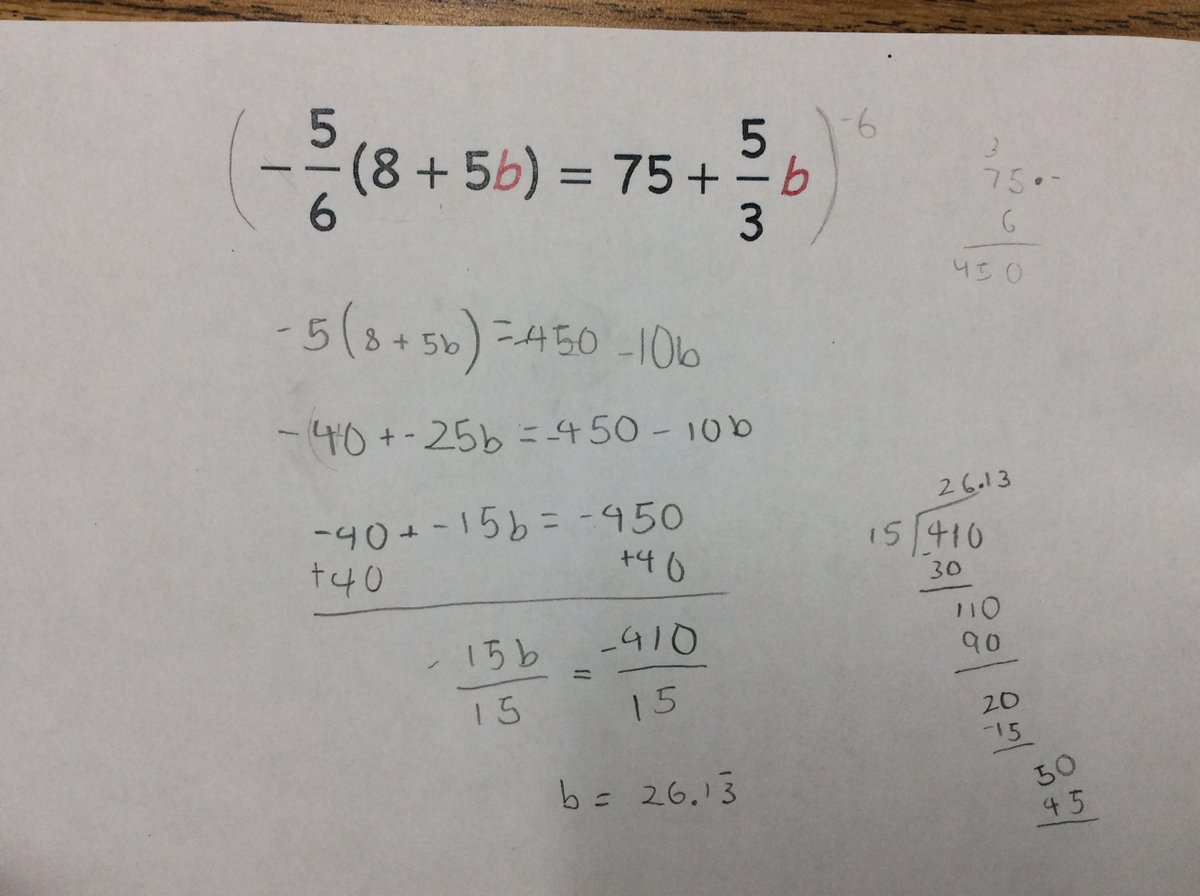Just be explicit about the mistake…we scale both sides by -6, but the left side ends up only getting scaled by 6. The fractions seem sort of incidental to this one. Maybe this is related to the thing where multiplying by a negative feels like it should make the stuff negative, rather than giving everything its opposite sign?

To what extent is this an equations mistake vs a negatives mistake?Lots going on here. The idea that we’re scaling both sides vs. just the fractions is tricky it seems. Clearly fractions make an equation tricky to solve as it presents a number of conceptual issues. To what extent is it helpful for a teacher to understand anything about how kids handle equations with fractions besides “with difficulty”? It seems like all the interesting choices to make when handling equations with fractions are about finding ways to transform that equation so that we can avoid those fractions.There’s an interesting choice to make about whether you try to multiply both sides of an equation by 2 or by -2. What makes that interesting is again that multiplying by a negative introduces conceptual issues that aren’t introduced by x2. I feel like I’m getting close to saying that, as a matter of coaching, it makes sense to encourage kids to scale equations by positive numbers whenever possible. (Which, I think, is always.)

Micro-skills involved: What do you choose to scale each side of the equation by? When do you choose to scale each side of the equation? (Do you distribute first, or scale first?)

***

This was one of mine that I wrote about elsewhere:

Yesterday I gave students a no-grades quiz in algebra. A student who, I had been told at the start of the year, frequently struggles in math, has been having a lot of success lately. She knew exactly how to handle both of the systems of equations that were on this short quiz, but she got stumped at one of the resulting equations:$-1.7x = 4.3x + 3.6$

I didn’t know what to say when she got stuck, exactly, but I was fairly confident that this was an example of a micro-skill that she was missing.

She and I agreed that she’d like me to write a little example on the side of her page, so I wrote this:$-2x = 5x + 7$

[I drew some arrows going down from each side labeled “+2x.”]$0 = 7x + 7$

My student read the example and then exclaimed (in a way I can only describe as “joyous”), Oh wait, you can make 0 there?!

Micro-skills: Knowing that you can make 0.

There seem to be a bunch of cases where you introduce and manage zeroes in solving equations. Another one of mine that I have rattling around in the archives:Check out the bottom right. This pair of students got frozen at y = 2y + 5 because they subtracted y from each side and then weren’t sure if they were allowed to end up with 0. (Two years ago I talked to these kids about this, so I’m not guessing from their work.) This seems identical to the situation that this year’s student.

The moral of the story to me is that making zeroes is something that students need “permission” to do. Not like permission from me, the mathematical authority. I just mean that it seems to be something that beginners need help realizing is a kosher move.

As long as this picture is here, another little decision that kids need to make (see top-right) is whether to add or subtract something when trying to use a balancing move.

Microskill: “making a zero” to decide whether to add/subtract something from each side of equation

Categories

## Constructing Trinomials – Where to go Next?

Hi everyone,

My name is Bryan Penfound. Awhile back I was asked if I would be interested in helping out at MathMistakes and I said yes not knowing how challenging this term would be for me. Now that I have settled in a little bit, I thought I was a bit overdue for a post, so here goes!

Recently while volunteering at a local high school in a grade 9 classroom, I had to opportunity to observe students’ answers to the following question: “Create a trinomial in the variable t that has degree 3 and a constant term of -4.”

Here are five of my favourite responses:I would love to get some discussion going. Choose one of the polynomials above and try to deconstruct what the student knows and what the student still has misconceptions about. What follow-up questions might you ask to learn more information about how the student is thinking? What follow-up questions might you ask to help with any current misconceptions?

Categories

## Using Desmos Activity Builder to Talk About Student WorkWhat do you notice about this student’s thinking? What do you wonder about it?

I made a little Desmos activity to see if it’s possible to use their activity builder to share and comment on student work. I asked people to circle something they noticed in this student’s work. Here is the overlay showing everything that everyone circled.I’m not sure what to make of all that overlaid, but I’m definitely interested. The written answers people offered were also really interesting. Here is a sampling:

Guest: “This is a common error for my students as well. They  do not recognize that this is a quadratic function and try to get a straight line.”

Kevin: “These don’t seem to be in any particular order.”

Lane: “Does he have an eraser? Does he get confused calculating with zero?  Does he know the shape of a parabola?  Does he know that a function cannot possible have one point on top of another? Does he sometimes get confused with x^2 and 2x?  Could he have analyzed his own mistakes with a calculator?  By not checking with a calculator will some of his errors snowball and cause further confusion?  Is the student feeling frustrated? I think it is good this student understands the choice of input does not have to be in a particular order.”

Jonathan: “I notice that there is a disconnect in the student’s knowledge of linear vs. quadratic equations. I wonder how come the student did not use any negative values in her table.”

While there were a lot of great observations, the one that stood out to me was that this student could probably learn to recognize that this sort of equation will produce a U shape. Knowing that this sort of equation produces a U will make it more likely that they will test negative x-values, or at least more reliably guess the rest of the shape. I agree: it seems as if this student is trying to fit a U-shaped function into a line-shaped paradigm.

What activity could we design that would help students like this one develop their thinking?

Inspired by Bridget, I put this together:In the Desmos activity, I asked if people could think of a way to improve my rough draft. Here were three responses that represent some of the variations people had:

On twitter, Bridget had a second idea for an activity that would help students like this one develop their thoughts.

To wrap things up, I shared a mockup of Bridget’s alternative activity and asked people what they thought about it.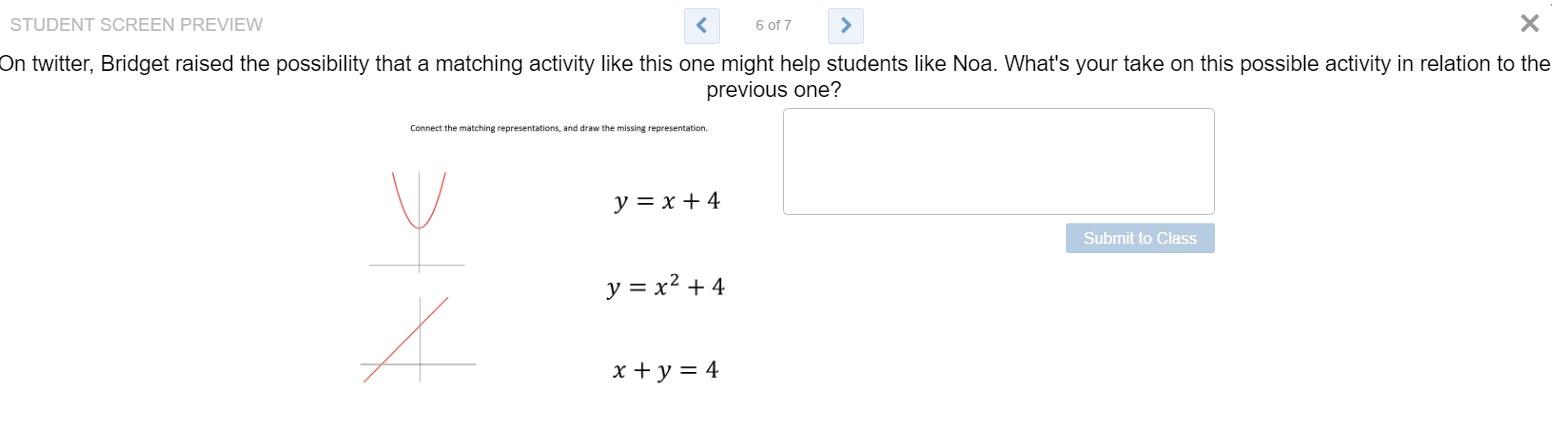Some selected responses:

Max: I prefer my version of the previous activity — this activity doesn’t invite students to consider why the parabola is symmetric — it’s easy enough to connect the linear and nonlinear representations and not confront the whys of the symmetry of the nonlinear representation. Maybe including y = x^3 + 4 as a third example (with both graph and equation provided) would support that sense-making?

Brian: I like the idea of having one more equation than graph. I’m also wondering about the choice to have two linear functions vs. one quadratic. This activity provides less structure than the previous since, to determine what the function’s graph looks like he would need to do it himself. The other provided the Desmos graph. On the other hand, this activity does provide the student with a more possibilities of visualizing the function, which could yield insight into how he’s thinking about the quadratic function.

Bridget: I wonder if the graphs should be discrete points instead of continuous. Not sure if it would make a difference or not. I also wonder if the missing representation should be another quadratic. I’m trying to consider if the connecting representations should include tables. I’m not sure…

Also, I think the previous slides connect more to the issue at hand. On an assessment-do you think this student could be given the equation y=x^2-3 and choose the correct graph from four multiple choice? I’m not sure…

Overall, this was fun! I’m excited to try it again.

Categories

## 5 out of 6 Books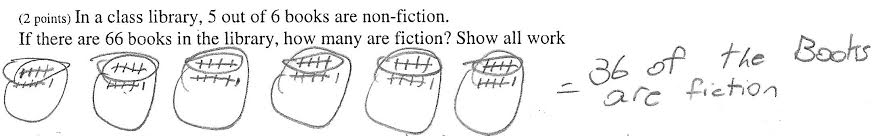At first, this is what I thought the student had done:

• First, the student drew six circles to represent “out of 6 books.”
• Then, they distributed, one-by-one, the 66 books into each of the 6 circles. (If they just put 11 in each, why tally them?)
• Then, the student searched for a way to represent the “5 out of” that are non-fiction.
• It follows that the remaining books are fiction. That makes six sixes, or 36 books.

But then Bridget and Julie came in with a fantastic, different interpretation. Their’s feels like an improvement on my first draft.

We then got to work trying to come up with some activities to address this work. Suppose that your class of 6th Graders try this problem, and a lot of your class has struggles that are similar to the work above. You’re planning tomorrow’s lesson. What activity would you begin class with?

This is what we came up with. Which of these activities do you think would be most helpful? Are there any changes you would make to any of them? Is there a combination and sequence of these activities that you think would work particularly well? (I took a shot at sequencing them below. Some details on activity structures are here.)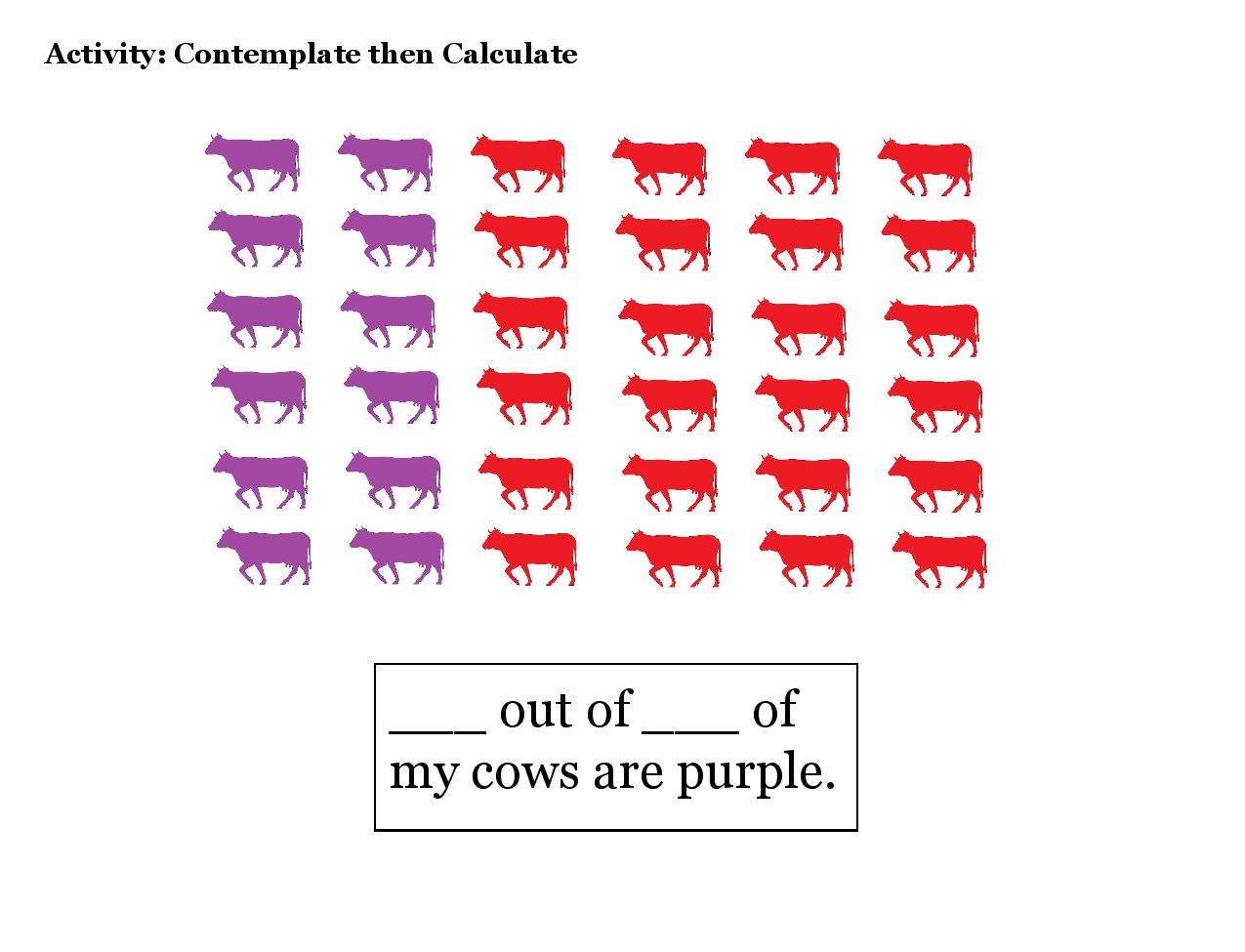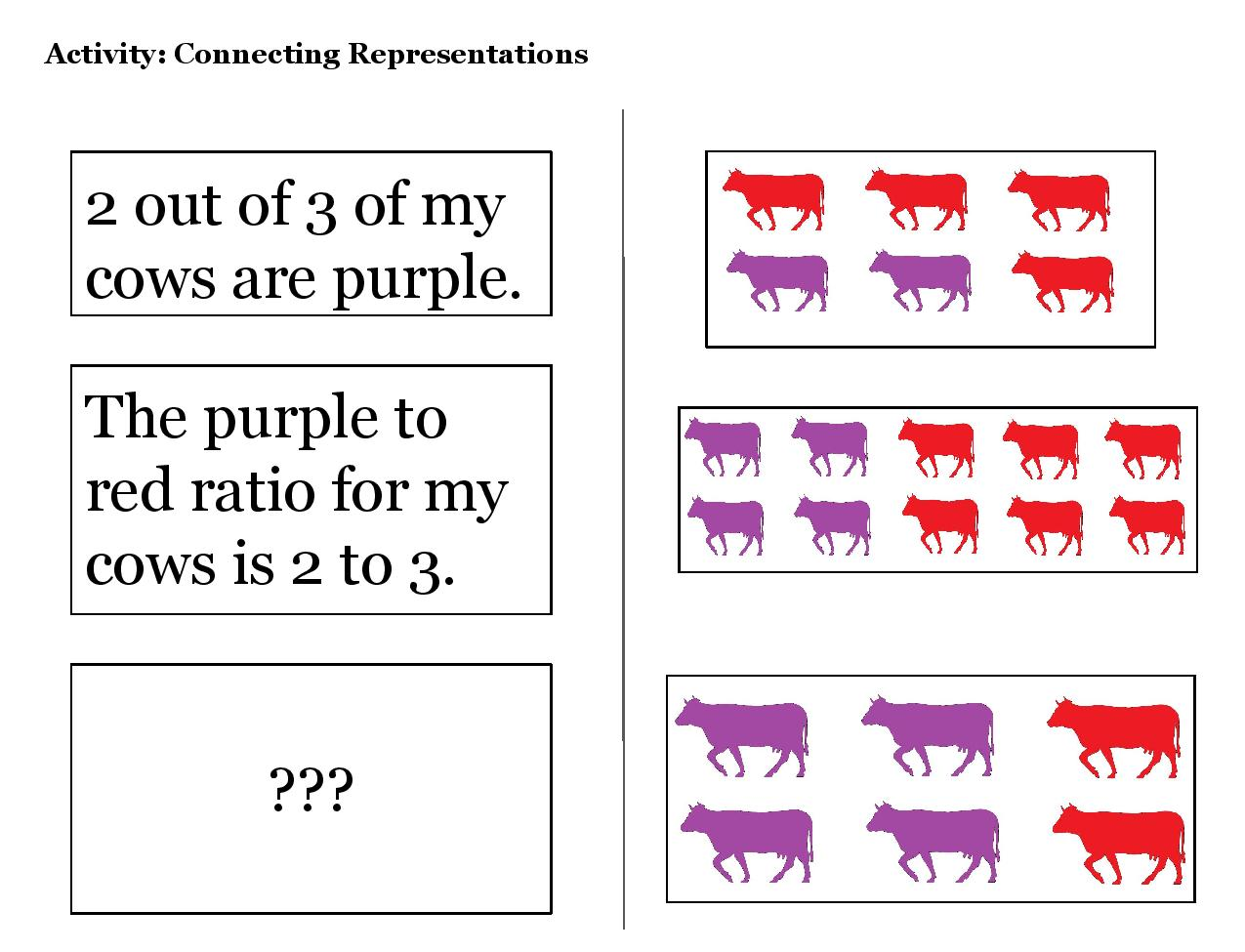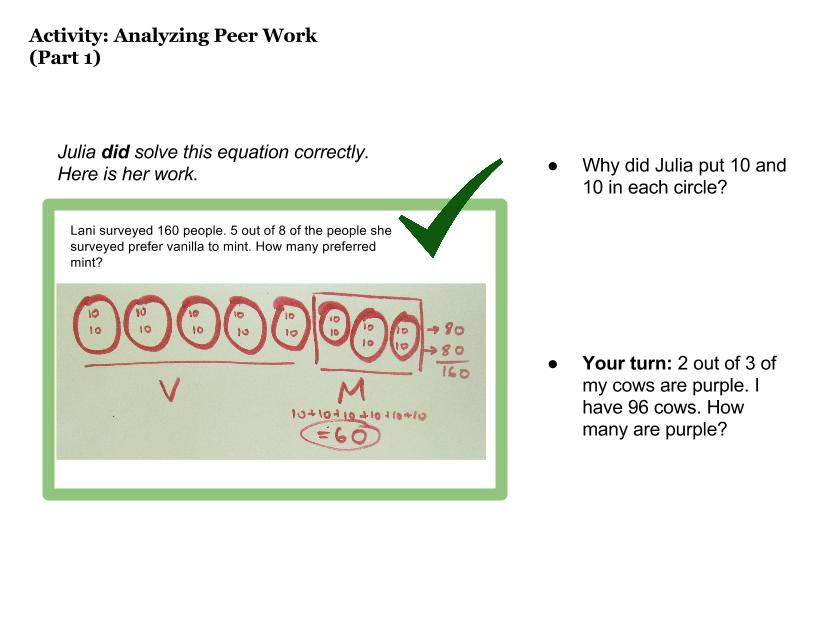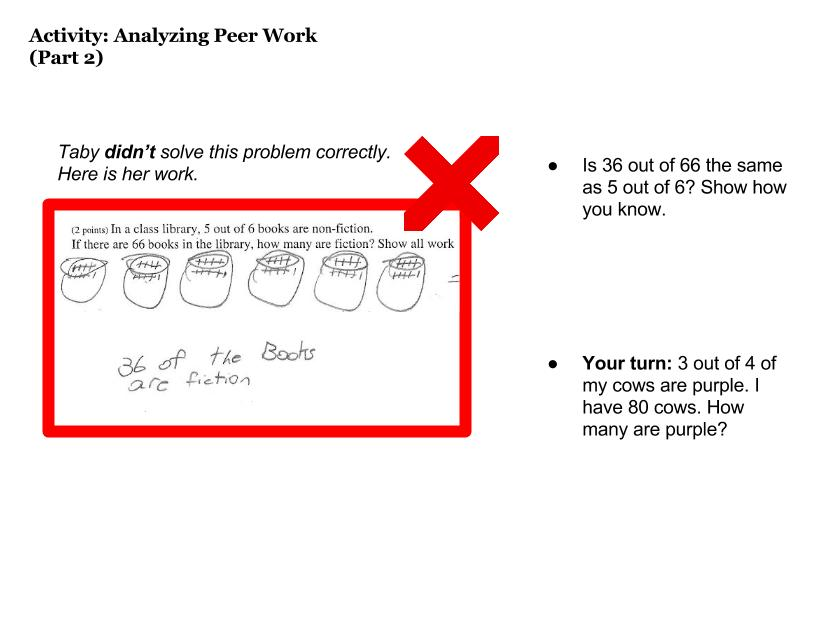Categories

## Which Activity Would You Choose?

In an earlier post, I shared Michael Fenton’s scenario and categorized the responses he got on twitter.

There were at least seven distinct responses that teachers offered to Fenton’s prompt. Wow! This makes me think two things:

1. Fenton’s scenario was so thought-provoking that it yielded an amazing variety of responses.
2. How come there was so much disagreement about how to act in this scenario?

Part of the disagreement, I think, comes from what went unspoken in Fenton’s mistake. We didn’t know if this mistake was shouted on in a discussion or found on a piece of paper. We don’t know if this is one of those times when we can afford to have a one-on-one conversation with a kid in response to her mistake, or if our response will be scrawled on her paper and returned. Was this a common error, or an isolated mistake? Could our response be an activity for the class instead of a chat?

While one-on-one conversations are crucial in teaching, they are hard to talk about. By their nature, they’re improvisational and somewhat unstructured. I’d also argue that opportunities for one-on-one conversations can be rare, and they get rarer as the number of students in your class grows larger.

Revising the Scenario

So let’s add some details to Fenton’s scenario. This was a mistake in an Algebra 1 class. Smart kids, thoughtful teacher, but when she collects papers after an ungraded check-in she finds that about half her class made Fenton’s mistake. Oh no! She decides that she’s going to launch class the next day with a brief activity to help advance her kids’ thinking.

Her first idea is to try a string of equations. She has three different drafts. Which one would you choose, and why?

Equation String 1Equation String 2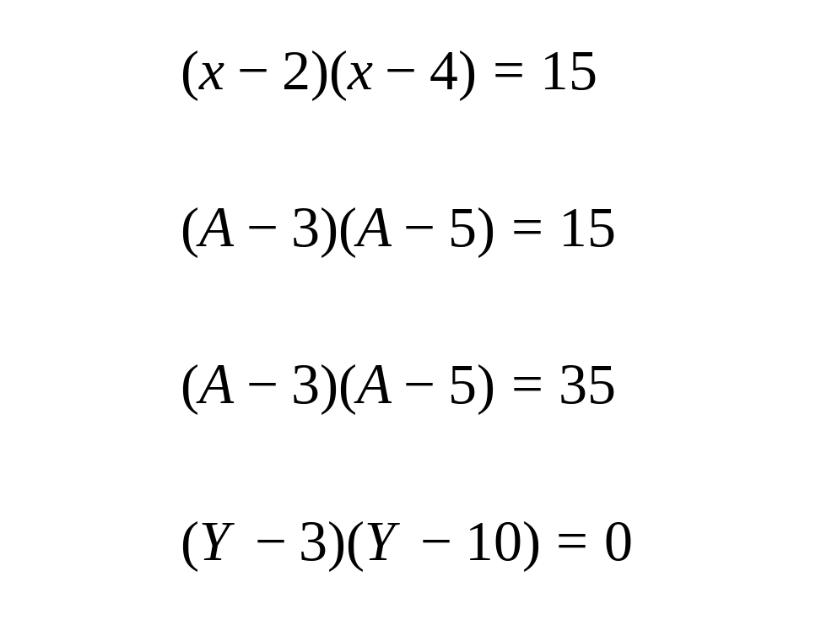Equation String 3Other Activities

Then, she has some other ideas. Maybe equation strings aren’t the right move? She comes up with three other activities: Working With Examples, Which One Doesn’t Belong and Connecting Representations.

Working With ExamplesConnecting RepresentationsWhich One Doesn’t Belong?Commentary

The meta-question here is about the conversation. Can we have a conversation with so many options? I don’t know. I worry that maybe I should have just limited discussion to the equation strings.

What would do?

My first reaction is that I like the equation strings, because it most directly gets at the issue of overextending the zero-product property to other equations.

But what I really want to do is lay out a sequence of 3-4 activities that I could do in sequence to develop this idea for a class.

And do I know enough to answer that question? Wouldn’t that depend on the math that we’d already studied and the math that’s coming up next?

Do we learn anything from thinking about these questions?

Categories

## How Teachers Would Respond to A Quadratics Mistake

Lots of responses to this great tweet. I wanted to understand the themes in what people were replying, so I went through everything and tried to summarize it here.

Response #1: Check Your Work, Start a Conversation

Response #2: Just Check Your Work (No Conversation Mentioned in Tweet)

Response #3: Explain the Zero Product Property

Response #4: Thinking About How to Teach the ZPP Unit

https://twitter.com/bowenkerins/status/741281577687240704

Response #5: Switch to a Graphical Context

Response #6: Ask for Explanations

Response #7: Run a New Activity with the Whole Class

I’m sure I didn’t capture everyone’s response, and I don’t know what any of this means. But there you go.

Categories

## Mistake Analysis + Practice

Here’s an activity I just drafted for my Algebra 1 class. I’m trying to help them get very comfortable working with the distributive property and fractions. Thoughts?